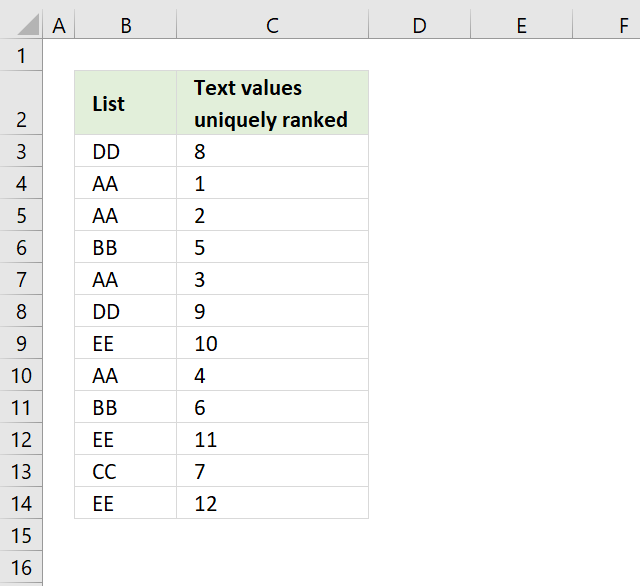Author: Oscar Cronquist Article last updated on November 16, 2018Question: How do I rank text cell values uniquely? If text values were sorted alphabetically from A to Z, the first text value would rank 1 and so on... Duplicate text values rank uniquely.

Formula in cell C3:

=COUNTIF(\$B\$3:\$B\$14,"<"&B3)+COUNTIF(\$B\$3:B3,B3)

Copy cell C3 and paste to cells below as far as needed.

### How does this formula work?

=COUNTIF(\$B\$3:\$B\$14,"<"&B3)+COUNTIF(\$B\$3:B3,B3)

Step 1 - Calculate rank

The COUNTIF function is an incredibly versatile function, in this case, instead of counting values based on a condition we simply check if a value is smaller or larger than the others.

Remember, we are using text values so the function returns a rank number based on the position if the list were sorted alphabetically.

COUNTIF(\$B\$3:\$B\$14,"<"&B3)

becomes

=COUNTIF({DD, AA, AA, BB, AA, DD, EE, AA, BB, EE, CC, EE}, "<"&DD)

and returns 7. 7 values are sorted before DD, as if they were sorted from A to Z.

The problem is that value DD has a duplicate and we need to make sure that the duplicate doesn't get the same number as the first instance.

Step 2 - Count current value and prior instances of current value

COUNTIF(\$B\$3:B3,B3)

becomes

COUNTIF("DD","DD")

and returns 1.

Note that the cell reference \$B\$3:B3 expands as we copy the cell to cells below.

Step 3 - Add values

COUNTIF(\$B\$3:\$B\$14,"<"&B3)+COUNTIF(\$B\$3:B3,B3)

becomes

7 + 1 and returns 8 in cell C3.

Final notes

Select B3 and use "evaluate formula" to see how the next cell calculates rank. Click "Evaluate" button and excel calculates cell formula step by step.